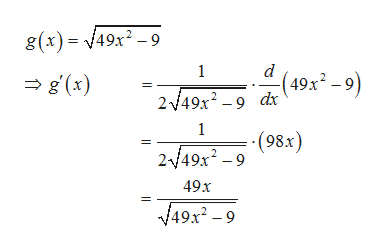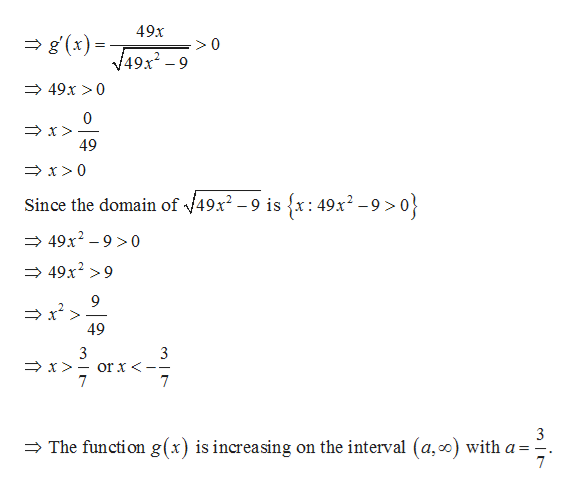# Let g(x)= square root49x2 − 9The function g is increasing on the following interval(s):  (−∞, ∞)(−∞, a)    (−∞, a](a, ∞)[a, ∞)(−∞, a) ∪ (b, ∞)(−∞, a] ∪ [b, ∞)(a, b)(a, b][a, b)[a, b]None a = b = g is decreasing on the following interval(s):(−∞, ∞)(−∞, c)    (−∞, c](c, ∞)[c, ∞)(−∞, c) ∪ (d, ∞)(−∞, c] ∪ [d, ∞)(c, d)(c, d][c, d)[c, d]Nonec = d =

Question
39 views
Let g(x)=
 square root 49x2 − 9

The function g is increasing on the following interval(s):

(−∞, ∞)
(−∞, a)

(−∞, a]
(a, ∞)
[a, ∞)
(−∞, a) ∪ (b, ∞)
(−∞, a] ∪ [b, ∞)
(a, b)
(a, b]
[a, b)
[a, b]
None

a =
b =

g is decreasing on the following interval(s):

(−∞, ∞)
(−∞, c)

(−∞, c]
(c, ∞)
[c, ∞)
(−∞, c) ∪ (d, ∞)
(−∞, c] ∪ [d, ∞)
(c, d)
(c, d]
[c, d)
[c, d]
None

c
d

check_circle

Step 1

Find the first derivative of the given function as follows.help_outlineImage Transcriptioncloseg(x)/49x2-9 d 1 (49к? -9) g(x) 249x29 dr 1 -(98x) 2/49x2 -9 49x 49x2 -9 fullscreen
Step 2

For g(x) to be increasing g’(x) has to be...help_outlineImage Transcriptionclose49x g(x) 49x2-9 49x >0 0 49 x 0 Since the domain of V49x2 - 9 is {x: 49x2-9 >0} 49x2 90 49x29 9 49 3 x or x < 7 3 _ The function g(x) is increasing on the interval (a,c0) with a 7 fullscreen

### Want to see the full answer?

See Solution

#### Want to see this answer and more?

Solutions are written by subject experts who are available 24/7. Questions are typically answered within 1 hour.*

See Solution
*Response times may vary by subject and question.
Tagged in

### Functions